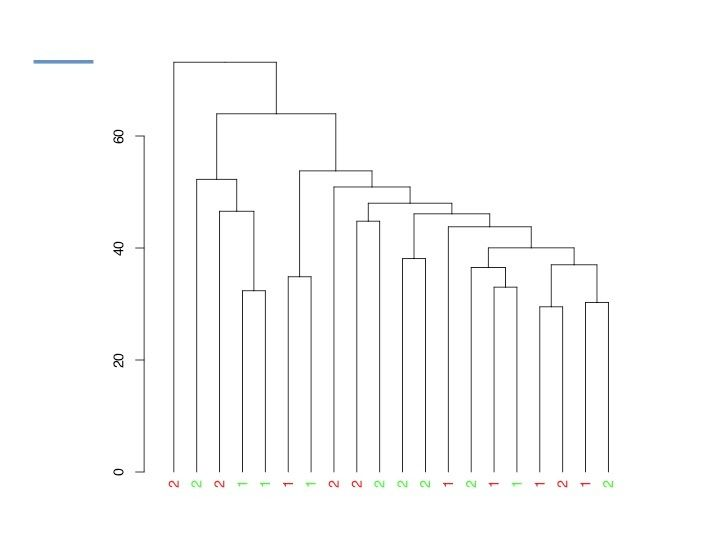Question: Alternative microarray analysis
0
alisce840 wrote:

I am analyzing 20 Agilent microarrays from humans (20 subjects, two conditions) and yet there is no differentially expressed gene after adjusting for multiple testing (BH). I used Limma in R, tried different background correction as well as normalization methods (within and between arrays), I also tried to remove the least expressed probes to try to boost the signal. All the array quality checks looked fine.

The question is, if there are no significantly differentially expressed genes, what can be done? Has anyone ever tried to get some results out of this situation or has a good paper/link to suggest?

Thanks all!

limma microarray R • 1.7k views
modified 5.5 years ago by Manvendra Singh2.1k • written 5.5 years ago by alisce840

How is the correlation between biological replicates ?

There are no biological replicates, let's say I have condition A on 10 subjects and condition B on the other 10

you can always look at the fold change values.

but they need to be significant, no?

Variance would be very high within the groups thats why you do not get significant DEGs between the groups, so better to try out as I suggested in the answer below,

lets see what happens then.

2
Manvendra Singh2.1k wrote:

Sometimes, It happens with large cohort of samples.

best to look into row-wise z-scores to see the sample wise alterations in gene expressions.

or

you have normalized expression values for each sample, you can take a row wise mean and calculate relative expression value for each gene in each sample, calculate spearman's correlation between the samples, cluster them and see how controls and cases are clustering.

if there are both controls and cases in single cluster then thats the reason of high variance within the group when you calculate DEGs.

better would be to consider only those clusters which contain either cases or controls, and make DEG analysis via limma by grouping them independently.

and then after may be to compare each cluster with each cluster and see overlapping or unique genes and so on you can play with data.

hth

Thank you for your suggestion, but I don't understand the procedure, although I got the concept.

What do you mean to calculate relative expression value for each gene, maybe to subtract the row wise mean from the gene expression value? Guess in this case we're talking about A values and not M values. Also, do you mean computing Spearman correlation between each couple of subjects? And then use all (above 40.000) probes to cluster subjects or just a sample?

1

do not substract, just divide

e.g. suppose if you have a dataframe (df) where row.names are genes and col.names are samples then in R

```######## load some libraries

library(plyr)

library(limma)

library(genefilter)

###### if data is not properly normalized

df=normalizeQuantiles(df, ties=TRUE)

###### calculate row mean

mean=apply(df, 1, mean)

###### relative expression
df.rel=df/mean
cor=cor(df.rel, method="spearman")

##### draw a dendrogram to see how it looks like

############################ more efficient way is to select top genes which shows more std.deviation within  ###  dataframe

percentage<-c(0.900) ###### selecting 0.1%
sds<-rowSds(df) ######## calculating std.deviation
sel<-(sds>quantile(sds,percentage)) ##### top deviating genes
set<-df[sel, ] ###### assigning to new set

####### clustering
distmeth<-c("euclidian")
D<-dist(t(set), method=distmeth)
treemeth<-c("average")
hc<-hclust(D, method=treemeth)
plot(hc)

####### see how it looks like

####### or you have your new dataframe named as "set" you can make heatmap cluster them with spearman's correlation or again calculate relative enrichment and see how many major clusters you are getting in your dataframe```

HTH

Thank you very much for your help.

See the dendrogram, where the color represents control and case, while the numbers 1 and 2 represents the two different batches. The arrays where done in two tranches at different times, but I corrected for this in with Combat. I also tried not using combat and just including the batch as a variable in the model (same result).

I also tried to double the sample size (copied control and cases, so I get twice the arrays) just to see if the low p.values were due to sample size. And in fact I get significant adjusted p values this way, and the significant probes overlap the top ones of the standard analysis. This showing that the problem relies in the small sample size.

But still I don't know how to deal with this - of IF there is something to do about it.

Following your suggestion and analyzing only clusters with either control or cases, well, there aren't any, as control and cases are spread out so homogeneously.